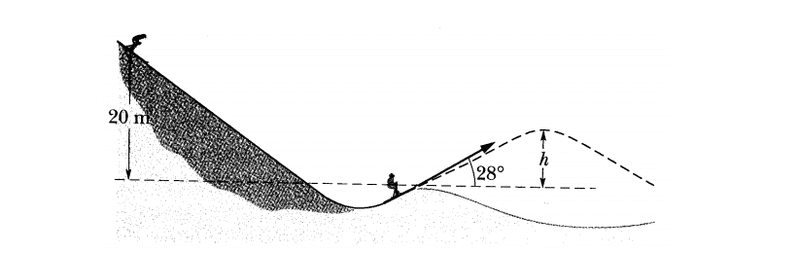# How to calculate the height of a skier

• JiJiasd
Then use this velocity to find the height at the top of the hill using the formula h=v2sin2∅/2g.In summary, the problem involves calculating the height of a skier in a figure using potential and kinetic energy equations, without friction. The formula used is h = v^2 * sin^2(θ)/2g, where v is the velocity at the launch point. It is important to use the correct component of speed when calculating h and to not mix up the specific height in the problem with the generic h in the equation.

## Homework Statement

I want to calculate the height h of the skier in this figure## Homework Equations

potential energy and kinetic energy and there is no friction

## The Attempt at a Solution

mgh = 0,5 *m*v^2
v=√ (2*g*h)

Now calculate the h when he goes up

mgh= 0,5 *m*√ (2*g*h)^2

Am I doing right ?

Thanks

[/B]

JiJiasd said:
Now calculate the h when he goes up

mgh= 0,5 *m*√ (2*g*h)^2
What you got is an identity. You need more information of the person on the top of the hill to get his condition, such as velocity~

mgh = 0,5 *m*v^2
v=√ (2*g*h)

This is right but after this, only calculate with its vertical component (v*sin(28 deg)), when calculating h.

tommyxu3 said:
What you got is an identity. You need more information of the person on the top of the hill to get his condition, such as velocity~
These informations are enough, you don't need more!

You only got an identity, because you didnt calculate with the right component of speed.

thank you all

You need to be careful with 'h'. There is a specific height in the problem which is labelled h, and there is the generic h in the equation gh=v2/2. Don't get them mixed up.
First, find the velocity at the launch point.